Next: Parallel version of ALIAS-Maple Up: ALIAS-Maple Previous: Univariate and parametric polynomial

• La page de J-P. Merlet
• La page Présentation de HEPHAISTOS
• La page "Présentation" de l'INRIA

Subsections

# Reusing a compiled program: the Restart procedure

It may be possible to restart a procedure with new parameters (usually a new search space) and reusing an already existing executable (hence it is not necessary to create a new executable). This is done with the Restart procedure that takes as first argument a string that indicate which procedure should be restarted and as following arguments new parameters for the procedure. See the on-line help to know which procedures can be restarted.

Note however that as soon as you have defined the ALIAS/ID string before generating an executable it is necessary to reset this string to the same name before using the Restart procedure to re-run the same executable

# Expression conversion: Convert_Frac_Cons

ALIAS uses a special mechanism to convert an expression into an equivalent C++ sentence that can be interval evaluated. This conversion is based on the conversion of the expression into a string in which all mathematical functions are substituted by their interval equivalent. This causes a problem if rational numbers are present in the expression as rational such as 1/6 will lead to 0 in C++. This procedure returns a string that describes the interval equivalent of the expression in which rational such as 1/6 as 1./6. and constant such as Pi have been converted into their C++ equivalent. For example


Convert_Frac_Cons(1/6*Sin(v_IS(1))+Pi+2/3*Cos(v_IS(2)));

1./6.Sin(v_IS(1))+BiasPi+2./3.*Cos(v_IS(2))


# Multiple occurrences of variables: MultipleOccurence

This procedure takes as input an expression and a list of variables and returns 1 if there are multiple occurrences of at least one variable. For example


MultipleOccurence(x^2+y^2-1,[x,y])

will return 0 while

MultipleOccurence(x^2+x+y^2-1,[x,y]);

will return 1.

This procedure is useful to test the transformation of an expression: is there is no multiple occurrences of variables in an expression, then the interval evaluation of this expression will be exact in the sense that it will return the exact lower and upper bounds of the expression for given intervals for the variables (which is not the case when multiple occurrence of variables appear in an expression).

# Mathematical functions in an expression

The procedure Math_Func allows one to obtain a list of mathematical functions (in the Maple sense) and of their power in a given expression. For example

Math_Func([(sin(x)^2+cos(x)^3)/x+sin(x)^2*cos(x)^3+1/sin(x)^2])

returns in the global variable ALIAS_MATH_FUNC the list
                                3        2     1
[cos(x) , sin(x) , -------]
2
sin(x)

If these terms appears more than once in the expression the MakeF procedure (see section 2.1.1) will generate a code where they are evaluated for each occurrence, which is costly. The ALIAS_FSIMPLIFY mechanism described in the MakeF section may be used to evaluate only once these expressions and substitute them by interval when calculating the interval evaluation. The end-user write its own ALIAS_FSIMPLIFY procedure but the result of Math_Func may be used to write such a procedure. A typical ALIAS_FSIMPLIFY using this functionality is as follows:

ALIAS_FSIMPLIFY:=proc(fid,expr)
local aux,ai:
if type(expr,string) then
fprintf(fid,"INTERVAL_VECTOR ALIAS_S(%d);\n",nops(ALIAS_MATH_FUNC)):
for ai from 1 to nops(ALIAS_MATH_FUNC) do
aux:=Convert_Frac_Cons(Code(ALIAS_MATH_FUNC[ai],[x,y,z,w])):
fprintf(fid,"ALIAS_S(%d)=%s;\n",ai,aux):
od:
RETURN(0):
fi:
aux:=expr:
for ai from 1 to nops(ALIAS_MATH_FUNC) do
aux:=subs(ALIAS_MATH_FUNC[ai]=ALIAS_S(ai),aux):
od:
RETURN(aux):
end:

Note the use of the Code procedure to convert the expression into an interval equivalent.

# Ordering a list of variables

The procedure Sort_Variable sort a list of variable in decreasing order according to the number of occurrences of the variable in a given expression. This list may be used as the argument of the Maple procedure convert,horner to convert an expression into a form that is more convenient for interval evaluation. For example consider the expression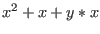: by using the procedure we get the sorted list [x,y] and


convert(x^2+x+y*x,horner,[x,y])

leads to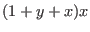while

convert(x^2+x+y*x,horner,[y,x])

leads to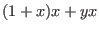which is less efficient. The number of occurrences of a variable may be found in the global variable ALIAS_OCCURENCES.

# Finding elementary components in a function

The procedure Decompose_Diff decomposes an expression in a list of elementary components i.e. powers of terms and functions. For example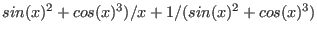will be decomposed into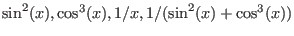. This procedure may be used to design a ALIAS_FSIMPLIFY procedure of MakeF so that the elementary components are evaluated only once even if they have multiple occurences in the expression. The list of elementary components may be found in the global variable ALIAS_TERMS. For a list of expression one has to use Decompose_Diff_List that will provide a list sorted by decreased order of length (as defined by the length procedure of Maple.

# Transformation of an expression: MinimalCout

It is well known that the interval evaluation of an expression is sensitive to the manner with which the expression is written. For example in term of interval analysis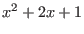is not equivalent to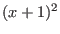as the interval evaluation of these two forms will usually be different. Unfortunately there is usually no known rule to determine which form will lead to the best interval evaluation of an expression (except if there is exactly one and only one occurrence of each variable in the expression). Hence ALIAS-Maple uses a set of heuristics to transform an expression into a form that leads usually to a good interval evaluation. The procedure MinimalCout takes as argument an expression and returns a mathematical equivalent of this expression but in a form that may be better for the interval evaluation.

Among the heuristics that are used in this procedure we use a conversion into Horner form. For a multivariate expression there are different Horner form according to the ordering of the variable. MinimalCout will consider all possible ordering of the variable to produce the best form but this may be computer intensive if there is a large number of variables. Hence all the ordering will be tested only if the number of variables does not exceed ALIAS/mincout with a default value of 8. Reducing the value of ALIAS/mincout will allow to reduce the computation time for calculating the best form for the expression as the possible expense of getting a less sharper interval evaluation. If the use of MinimalCout has to be avoided it is sufficient to set ALIAS/mincout to a negative value.

MinimalCout accepts an optional second argument which is a list of variables. The procedure will try find an expression that is the best with respect to this list.

# Newton scheme

We have a specific Maple implementation of the Newton scheme which is mostly be intended to be used in conjunction with the result of the solving algorithms of ALIAS-C++. The main purpose of this procedure is to allow to refine the accuracy of the result provided by the solving procedures of ALIAS-C++ which have an accuracy of double. It will allow to calculate the roots of a system with an accuracy that is specified in term of number of digits. For example when specifying an accuracy of 200 digits the result provided by this procedure (when it succeeded) will be a number with 200 digits, the last digit being guaranteed to be the correct digit for the solution.

Newton(Func,Vars,Init,Maxiter,Acc)

where
• Func: a list with the system of equations to be solved (which must be square)
• Vars: a list with the name of the unknowns
• Init: a list for the initial guess. This list may be either an element of the list returned by the solving procedure of ALIAS (i.e. with 2 elements for each variable) or a list with one element for each variable
• Maxiter: maximum number of iteration allowed for the scheme
• Acc: accuracy for the solution: the Acc-th bit is guaranteed to be exact
This procedure returns:
• -4: number of Init element not compatible with the number of elements in Vars
• -2: not a square system
• -3: singular jacobian matrix
• -1: more than MaxIter iterations
• 1: success
and the estimation of the solution in ALIAS_Newton.

Clearly this procedure may fail to return a correct result in some cases: for example if the correct result of an univariate equation is 2 it may happen that the numerical Newton scheme always converge to 1.999999999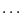.

# The Bound_Distance procedure

This procedure is specific for systems of distance equations (see section 3.3.2.1) for determining an initial search domain. It may determine either determine an initial guess for the input intervals or to improve a given initial guess.

The syntax is:


Bound_Distance(Func,Var,Result)

where Func is a list of distance equations, Var a list of variable name and Result is the initial search domain.

An optional fourth argument of this procedure is an initial guess for the search domain. The procedure will return:

• -1: there is no solution to the system of equations
• 0: the procedure has not been able to find an initial search domain or to improve the initial guess
• 1: the procedure has been able to determine an initial guess or to improve the initial guess

# Drawing for non-0 dimensional systems: DrawND

The ALIAS-Maple solving procedure allows to compute an approximation of the solutions of non-0 dimensional system as a a set of boxes (see section 3.4). The procedure DrawND allows on to visualize 2D or 3D cross-sections of the result:


DrawND(File,Nb,PLotL,Compact)

where
• File: is the name of the result file provided by the solving algorithm
• Nb: the number of variables in the result file
• PlotL: a list of number that indicates which variable will be plotted. This list must have 2 or 3 elements. For example if the list if [1,10], then a 2D plot with variables 1, 10 will be returned while if the list is [1,2,3] a 3D plot will be returned
• Compact: if this integer is set to 1 the procedure will try to regroup boxes in the file in order to reduce the plotting time
The procedure returns a plot structure that may be visualized with the Maple procedure display.Next: Parallel version of ALIAS-Maple Up: ALIAS-Maple Previous: Univariate and parametric polynomial